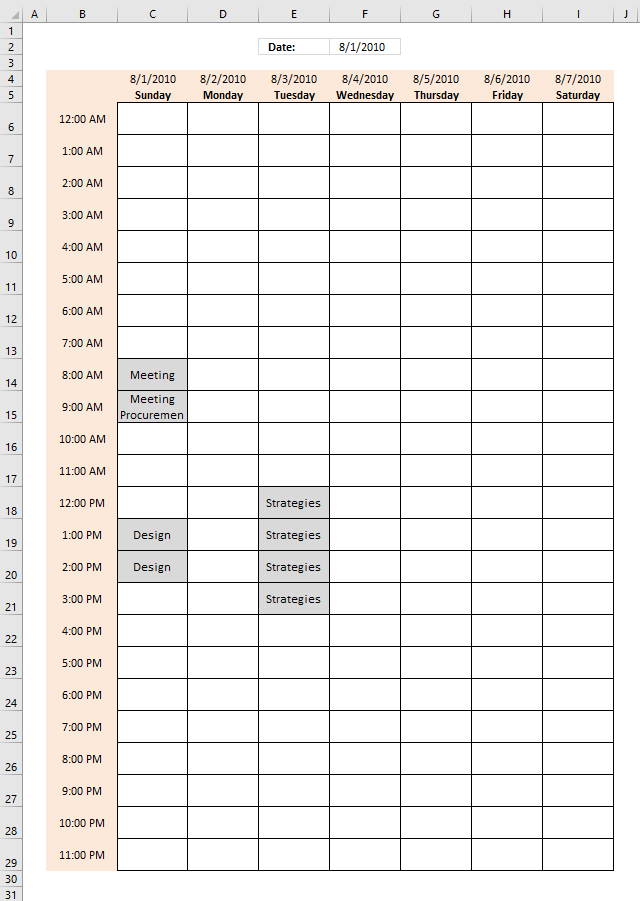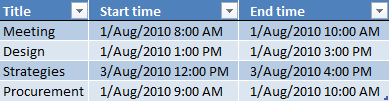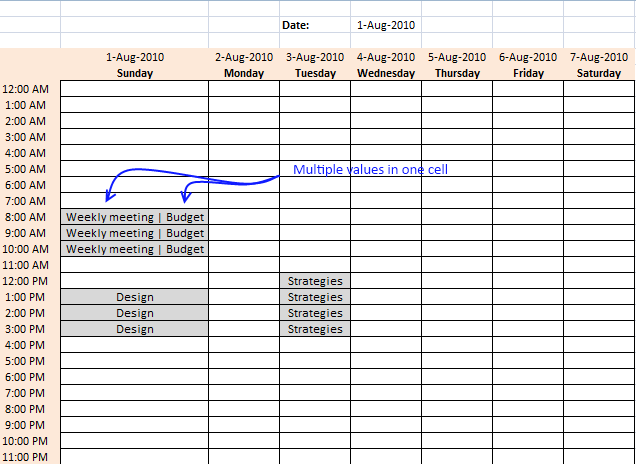Author: Oscar Cronquist Article last updated on October 23, 2019In this post I am going to add one more function to the weekly schedule I built in a previous article, an array formula allows you to populate cells with information from a schedule sheet.

The first part in this article demonstrates an array formula that contains the TEXTJOIN function, the second part in this post shows you how to show multiple events in a cell with the help of some vba code in case your Excel version doesn't contain the TEXTJOIN function.

You can easily adjust the height of the cells if you want to populate more than 2 events in a single cell.

Here is a picture of the schedule sheet:From the above picture we see that:

On the 1 of August from 8:00 AM to 10:00 AM the word "Meeting" will populate two cells on weekly schedule.

On the 1 of August from 9:00 AM to 10:00 AM the word "Procurement" will populate one cell on weekly schedule, shared with "Meeting"

On the 1 of August from 1:00 PM to 3:00 PM the word "Design" will populate two cells on weekly schedule.

On the 3 of August from 12:00 PM to 4:00 PM the word "Strategies" will populate four cells on weekly schedule.

Now let us see what happens if we change the date in cell F2 to 9-Aug-2010.

Formula in C4:

=\$F\$2-WEEKDAY(\$F\$2,1)+1

### Explaining formula in cell C4

#### Step 1 - Return weekday number

The WEEKDAY function converts a date to a weekday number from 1 to 7 based on when week starts.

WEEKDAY(\$F\$2,1)

becomes

WEEKDAY(40391,1)

and returns 1.

#### Step 2 - Calculate first date in week

\$F\$2-WEEKDAY(\$F\$2,1)+1

becomes

40391-1+1

and returns 40391.

If your week starts on a Monday then change the formula to \$F\$2-WEEKDAY(\$F\$2,1)+2

Formula in D4:

=C4+1

Copy cell C5 and paste it into cells E4:I4

Array formula in C6:

=TEXTJOIN(CHAR(10),TRUE,IF(((C\$4+\$B6)>=Table1[Start time])*((C\$4+\$B6)<Table1[End time]),Table1[Title],""))

Copy cell C6 and paste it into cell range C6:I29.

### Explaining formula in cell C6

The array formula uses cell references that points

#### Step 1 - Identify events based on date and time

Cell reference C\$4 is locked to row 4, the column reference changes only when the cell is copied to another column, this makes sure that the formula only gets values from row 4 (dates).

Cell reference \$B6 is locked to column B, the row reference changes only when the cell is copied to another row, this makes sure that the formula only gets values from column B (time value).

The formula checks if the current cell is inside one or more event ranges.

(C\$4+\$B6)>=Table1[Start time])*((C\$4+\$B6)<Table1[End time])

becomes

(40391)>=Table1[Start time])*((40391)<Table1[End time])

becomes

(40391)>={40391.3333333333; 40391.5416666667; 40393.5; 40391.375})*((40391)<{40391.4166666667; 40391.625; 40393.6666666667; 40391.4166666667})

and returns

{0;0;0;0}. This means that 8/1/2010 12:00 AM has no events scheduled.

#### Step 2 - Convert array to event name if equal to 1

The IF function has three arguments, the first one must be a logical expression. If the expression evaluates to TRUE (1) then one thing happens (argument 2) and if FALSE (0) another thing happens (argument 3).

IF(((C\$4+\$B6)>=Table1[Start time])*((C\$4+\$B6)<Table1[End time]),Table1[Title],"")

becomes

IF({0;0;0;0},Table1[Title],"")

becomes

IF({0;0;0;0},{"Meeting";"Design";"Strategies";"Procurement"},"")

and returns

{"";"";"";""}.

#### Step 3 - Concatenate values

The TEXTJOIN function uses CHAR(10) as a delimiting character, this will display each event name on a new row in one cell.

TEXTJOIN(CHAR(10),TRUE,IF(((C\$4+\$B6)>=Table1[Start time])*((C\$4+\$B6)<Table1[End time]),Table1[Title],""))

becomes

TEXTJOIN(CHAR(10),TRUE,{"";"";"";""})

and returns "" (nothing) in cell C6.

### Conditional formatting

I highlighted populated cells using a conditional formatting formula:

=C6<>""

### Get the Excel filePopulate-time-ranges-in-a-weekly-schedulev2.xlsx

### Populate multiple cell values in a single cell in a weekly schedule (vba)

What should I change if I have two different which start and end the same date time ? As it is now, only the first one is displayed. How I can divide them in order to see both of them?#### VBA code

```Function Lookup_concat(SearchDate As String, _
StartDate As Range, EndDate As Range, Return_val_col As Range)
Dim i As Long
Dim result As String
For i = 1 To StartDate.Count
If (StartDate(i, 1) * 1) <= SearchDate Then If (EndDate(i, 1) * 1) > SearchDate Then
result = result & Return_val_col.Cells(i, 1).Value & " | "
End If
End If
Next i
result = Left(result, Len(result) - 3)
Lookup_concat = Trim(result)
End Function
```

#### Where do I copy/paste vba code?

1. Press Alt-F11 to open visual basic editor
2. Select your workbook in project explorer
3. Press with left mouse button on Module on the Insert menu
4. Copy and paste the above user defined function
5. Exit visual basic editor

### Get the Excel filePopulate-time-ranges-in-a-weekly-schedule-version22.xlsm